# NCERT Solutions for Class 12 Mathemetics Chapter 12 - Linear Programming

##### Question 1:

Solve the following Linear Programming Problems graphically :
Maximise Z = 3x + 4y subject to the constraints :
x + y $\le$ 4, x $\ge$ 0, y $\ge$0.

The system of constraints is :
x + y $\le$ 4 ...(1)
and x $\ge$0. y $\ge$ 0 ...(2)
The shaded region in the following figure is the feasible region determined by the system of constraints (1) – (2).
It is observed that the feasible region OAB is bounded.
Thus we use Corner Point Method to determine the maximum value of Z, where :
Z = 3x + 4y ...(3)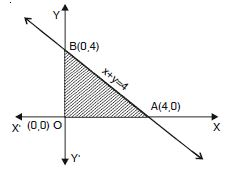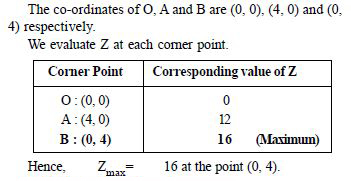##### Question 2:

Minimise Z = – 3x + 4y
subject to x + 2y $\le$ 8, 3x + 2y $\le$ 12, x $\ge$ 0, y $\ge$0.

The system of constraints is :
x + 2y$\le$ 8 ...(1)
3x + 2y$\le$ 12 ...(2)
and x $\ge$0, y$\ge$ 0 ...(3)
The shaded region in the following figure is the feasible region determined by the system of constraints (1) – (3).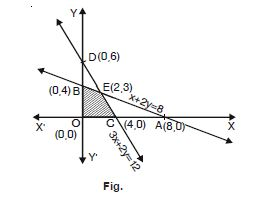It is observed that the feasible region OCEB is bounded.
Thus we use Corner Point Method to determine the minimum value of Z, where
Z= – 3x + 4y ...(4)
The co-ordinates of O, C, E and B are (0, 0), (4, 0), (2, 3) (on solving x + 2y = 8 and 3x + 2y = 12) and (0, 4) respectively.
We evaluate Z at each corner point.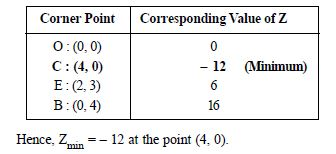##### Question 3:

Maximise Z = 5x + 3y
subject to 3x + 5y $\le$ 15, 5x + 2y $\le$ 10, x $\ge$0, y $\ge$0.

The system of constraints is :
3x + 5y $\le$ 15 ...(1)
5x + 2y $\le$ 10 ...(2)
and x $\ge$0, y $\le$ 0 ...(3)
The shaded region in the following figure is the feasible region determined by the system of constraints (1)–(3).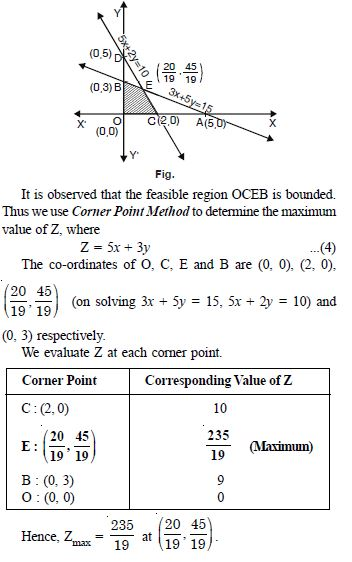##### Question 4:

Minimise Z = 3x + 5y
subject to x + 3y $\ge$3, x + y $\ge$2, x, y $\ge$0.

The system of constraints is :
x + 3y $\ge$ 3 ...(1)
x + y $\ge$ 2 ...(2)
and x, y $\ge$ 0 ...(3)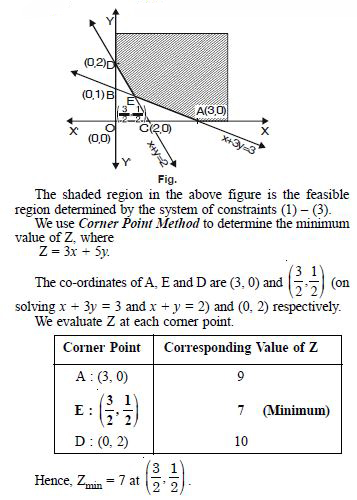##### Question 5:

Maximise Z = 3x + 2y
subject to x + 2y $\le$ 10, 3x + y $\le$ 15, x, y $\ge$0.

The system of constraints is :
x + 2y $\le$ 10 ...(1)
3x + y $\le$ 15 ...(2)
and x, y $\ge$ 0 ...(3)
The shaded region in the following figure is the feasible region determined by the system of constraints (1) – (3).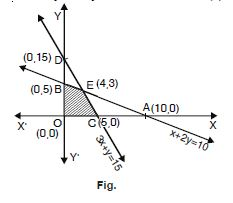It is observed that the feasible region OCEB is bounded.
Thus we use Corner Point Method to determine the maximum
value of Z, where :
Z = 3x + 2y ...(4)
The co-ordinates of O, C, E and B are (0, 0), (5, 0),
E (4, 3) (solving x + 2y = 10, 3x + y = 15) and (0, 5)
respectively.
We evaluate Z at each corner point.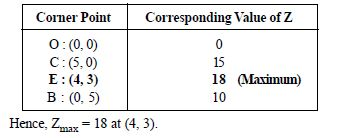##### Question 6:

Minimise Z = x + 2y
subject to 2x + y $\ge$3, x + 2y $\ge$6, x, y $\ge$0.
Show that the minimum of Z occurs at more than two points.

The system of constraints is :
2x + y $\ge$ 3 ...(1)
x + 2y $\ge$ 6 ...(2)
and x $\ge$ 0, y $\ge$ 0 ...(3)
The shaded region in the following figure is the feasible region determined by the system of constraints (1)–(3).
It is observed that the feasible region is unbounded.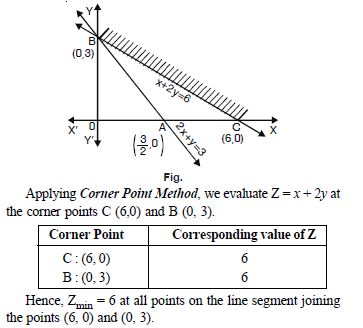##### Question 7:

Minimise and Maximise Z = 5x + 10y
subject to x + 2y $\le$ 120, x + y $\ge$60, x – 2y $\ge$0, x, y $\ge$0.

The system of constraints is :
x + 2y $\le$ 120 ...(1)
x + y $\ge$ 60 ...(2)
x – 2y $\ge$ 0 ...(3)
and x, y $\ge$ 0 ...(4)
It is observed that the feasible region CADE is bounded whose corner points are :
C (60, 0), A (120, 0), D (60, 30), (Solving x + 2y = 120 and x – 2y = 0) and E (40, 20) (Solving x + 2y = 60 and x – 2y = 0).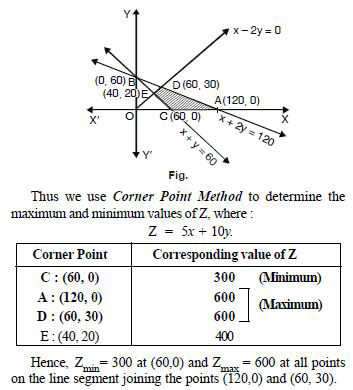##### Question 8:

Minimise and Maximise Z = x + 2y
subject to x + 2y $\ge$ 100, 2x – y $\le$ 0, 2x + y $\le$ 200; x, y $\ge$0

The system of constraints is :
x + 2y $\ge$ 100 ...(1)
2x – y $\le$ 0 ...(2)
2x + y $\le$ 200 ...(3)
and x, y $\le$ 0 ...(4)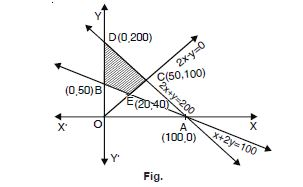The shaded region in the above figure is the feasible region determined by the system of constraints (1)–(4).
It is observed that the feasible region ECDB is bounded. Thus we use Corner Point Method to determine the maximum of Z,
where :
Z = x + 2y ...(5)
The co-ordinates of E, C, D and B are (20, 40)(on solving x + 2y = 100 and 2x – y = 0)
(50, 100)(on solving 2x + y = 200 and 2x – y = 0)
(0, 200) and (0, 50) respectively.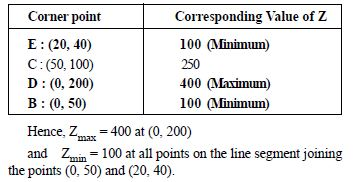##### Question 9:

Maximise Z = – x + 2y, subject to the constraints :
x $\ge$3, x + y $\ge$5, x + 2y $\ge$6, y $\ge$0.

The system of constraints is :
x ? 3 ...(1)
x + y ? 5 ...(2)
x + 2y ? 6 ...(3)
and y ? 0 ...(4)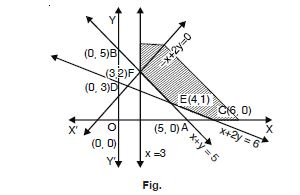The shaded region in the above figure is the feasible region determined by (1)–(4)
The corner points are C (6, 0), E(4, 1) and F (3, 2).
Applying Corner Point Method, we have :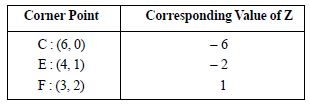It appears that Zmax = 1 at (3, 2).
But the feasible region is unbounded, therefore, we draw the graph of the inequality – x + 2y > 1.
Since the half-plane represented by – x + 2y > 1 has points common with the feasible region,
$\therefore$ Zmax $\ne$ 1.
Hence, Z has no maximum value.

##### Question 10:

Maximise Z = x + y subject to : x – y $\le$ – 1, – x + y $\le$ 0, x, y $\le$ 0.

The system of constraints is :
x – y $\le$ – 1 ...(1)
– x + y $\le$ 0 ...(2)
x, y $\ge$ 0 ...(3)
Draw the lines x – y = – 1 and – x + y = 0.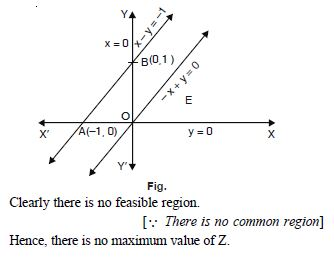##### Question 11:

Reshma wishes to mix two types of food P and Q in such a way that the vitamin contents of the mixture contain at least 8 units of vitamin A and 11 units of vitamin B. Food P costs ₹ 60/kg and Food Q costs ₹ 80/kg. Food P contains 3 units/kg of Vitamin A and 4 units/kg of vitamin B. Food Q contains 5 units/kg of Vitamin A and 2 units/kg of vitamin B. Determine the minimum cost of the mixture.

Let Reshma mix ‘x’ kg of food P and ‘y’ kg of
food Q.
Thus the LPP problem is as follows :
Minimize
Z = 60x + 80y
Subject to :
3x + 4y $\ge$ 8 ...(1)
5x + 2y $\ge$ 11 ...(2)
and x, y $\ge$ 0 ...(3)
The shaded portion represents region, which is unbounded.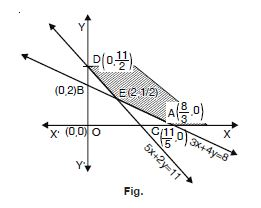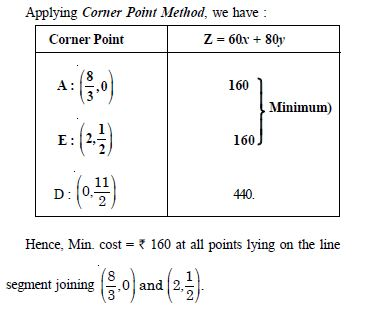##### Question 12:

One kind of cake requires 200g of flour and 25 g of fat and another kind of cake requires 100 g of flour and 50 g of fat.Find the maximum number of cakes which can be made from 5 kg of flour and 1 kg of fat assuming that there is no shortage of the other ingredients used in making the cakes.

Let ‘x’ and ‘y’ be the number of cakes of first and second kind respectively.
Thus the LPP problem is as follows :
Maximize Z = x + y
Subject to :200 x + 100y $\le$ 5000
? 2x + y $\le$ 50 ...(1)
25x + 50y $\le$ 1000 $\le$ x + 2y $\le$ 40 ...(2)
and x, y $\ge$ 0.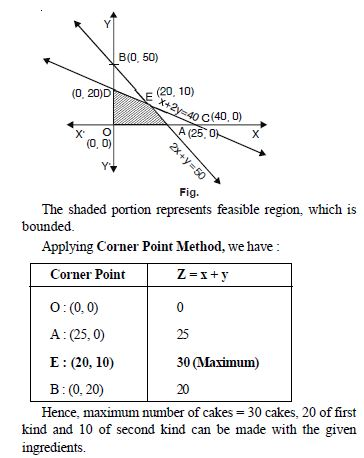##### Question 13:

A factory makes tennis rackets and cricket bats. A tennis racket takes 1·5 hours of machine time and 3 hours of craftman’s time in its making while a cricket bat takes 3 hours of machine time and 1 hour of craftman’s time. In a day,the factory has the availability of not more than 42 hours of machine time and 24 hours of craftman’s time.
(i) What number of rackets and bats must be made if the factory is to work at full capacity ?
(ii) If the profit on a racket and on a bat is ` 20 and ` 10 respectively, find the maximum profit of the factory when it works at full capacity.

Let the factory make ‘x’ tennis rackets and ‘y’ cricket bats.
We have :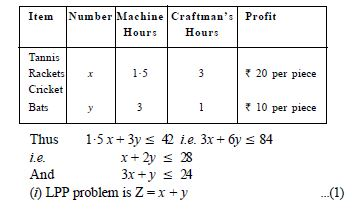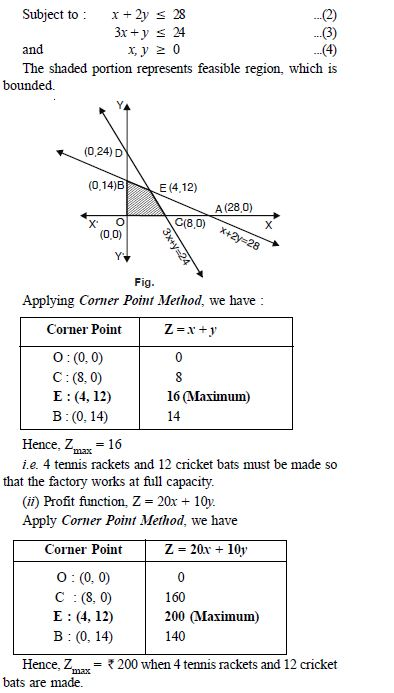##### Question 14:

A factory makes tennis rackets and cricket bats. A tennis racket takes 1·5 hours of machine time and 3 hours of craftman’s time in its making while a cricket bat takes 3 hours of machine time and 1 hour of craftman’s time. In a day,the factory has the availability of not more than 42 hours of machine time and 24 hours of craftman’s time.
(i) What number of rackets and bats must be made if the factory is to work at full capacity ?
(ii) If the profit on a racket and on a bat is ` 20 and ` 10 respectively, find the maximum profit of the factory when it works at full capacity.

Let the factory make ‘x’ tennis rackets and ‘y’ cricket bats.
We have :##### Question 15:

A manufacturer produces nuts and bolts. It takes 1 hour of work on machine A and 3 hours on machine B to produce a package of nuts while it takes 3 hours on machine A and 1 hour on machine B to produce a package of bolts. He earns a profit of ` 17·50 per package of nuts and ` 7.00 per package of bolts. How many packages of each should be produced each day so as to maximize his profit,if he operates his machines for at the most of 12 hours a day ? Formulate this mathematically and then solve it.

Let ‘x’ and ‘y’ be the number of packages of nuts and bolts respectively.
We have the following constraints :
x $\ge$ 0 ...(1)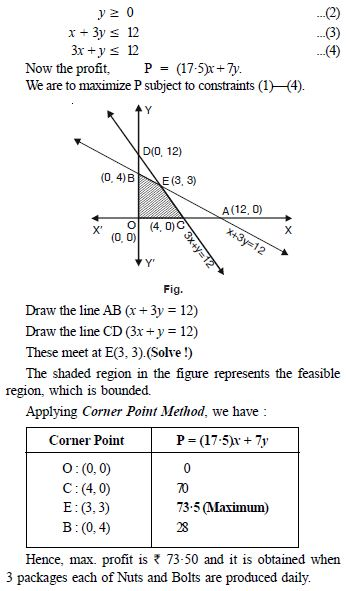##### Question 16:

A factory manufactures two types of screws, A and B. Each type of screw requires the use of two machines, an automatic and a hand operated. It takes 4 minutes on the automatic and 6 minutes on hand operated machine to manufacture a package of screws A, while it takes 6 minutes on automatic and 3 minutes on the hand operated machine to manufacture a package of screws B. Each machine is available for at the most 4 hours on any day. The manufacturer can sell a package of screws A at a profit of ` 7 and screws B at a profit of ` 10. Assuming that he can sell all the screws he manufactures, how many packages of each type should the factory owner produce in a day in order to maximise his profit ? Determine the maximum profit.

Let the manufacturer produce ‘x’ packages of Screws
A and ‘y’ packages of Screws B.
Thus the LPP problem is as follows :
Maximize : Z = 7x + 10y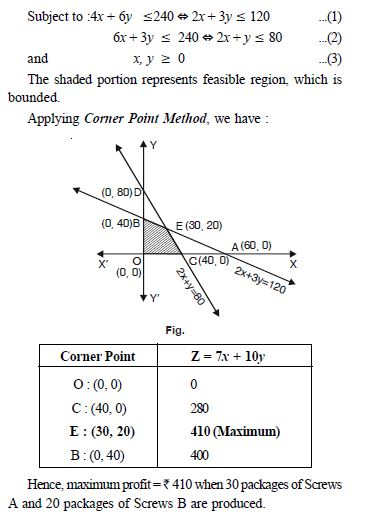##### Question 17:

A cottage industry manufactures pedestal lamps and wooden shades, each requiring the use of a grinding/cutting machine and 3 hours on the sprayer to manufacture a pedestal lamp. It takes 1 hour on the grinding/cutting machine and 2 hours on the sprayer to manufacture a shade. On any day, the sprayer is available for at the most 12 hours and the grinding/cutting machine for at the most 12 hours. The profit from the sale of a lamp is ` 5 and that from a shade is ` 3. Assuming that manufacturer can sell all the lamps and shades that he produces, how should he schedule his daily production in order to maximise his profit ?

Let the manufacturer produce ‘x’ Pedestal
lamps and ‘y’ Wooden shades everyday. Then the LPP
problem is as follows :
Maximize : P = 5x + 3y ...(1)
Subject to : 2x + y $\le$ 12 ...(2)
3x + 2y $\le$ 20 ...(3)
and x $\ge$ 0, y $\ge$0 ...(4)
The shaded portion represents feasible region, which is bounded.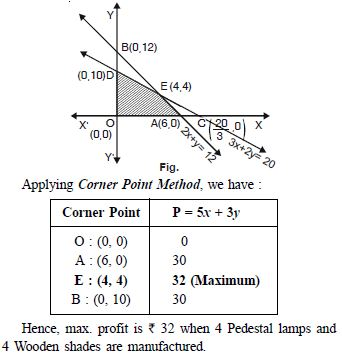##### Question 18:

A company manufactures two types of novelty souvenirs made of plywood. Souvenirs of type A require 5 minutes each for cutting and 10 minutes each for assembling. Souvenirs of type B require 8 minutes each for cutting and 8 minutes each for assembling. There are 3 hours 20 minutes available for cutting and 4 hours for assembling. The profit is ₹ 5 each for type A and ₹ 6 each for type B souvenirs. How many souvenirs of each type should the company manufacture in order to maximise the profit ?

Let the company manufacture ‘x’ Souvenirs of type A and ‘y’ Souvenirs of type B.
Then the LPP problem is as follows :
Maximize P = 5x + 6y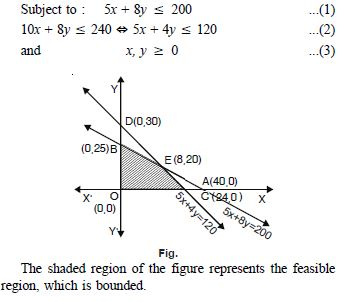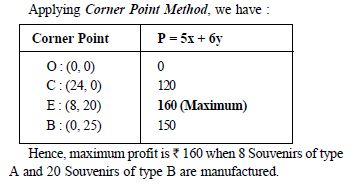##### Question 19:

A merchant plans to sell two types of personal computers–a desktop model and a portable model that will cost ₹ 25000 and ₹ 40000 respectively. He estimates that the total monthly demand of computers will not exceed 250 units. Determine the number of units of each type of computers which the merchant should stock to get maximum profit if he does not want to invest more than ₹ 70 lakhs and his profit on the desktop model is ₹ 4500 and on portable model is ₹ 5000.

Let the merchant stock ‘x’ Desktop computers and ‘y’ Portable computers.
Thus the LPP problem is as follows :
Maximize :
P = 4500x + 5000y
Subject to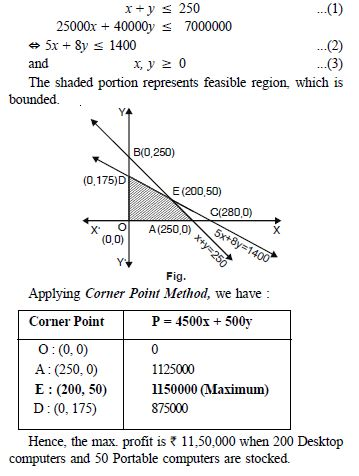##### Question 20:

A diet is to contain at least 90 units of vitamin A and 100 units of minerals. Two foods F1 and F2 are available. Food F1 costs ₹ 4 per unit food and F2 costs ₹ 6 per unit. One unit of food F1 contains 3 units of vitamin A and 4 units of minerals.One unit of food F2 contains 6 units of vitamin A and 3 units of minerals. Formulate this as a linear programming problem. Find the minimum cost for diet that consists of mixture of these two foods and also meets the minimal nutritional requirements.

Let diet contain ‘x’ units of food F1 and ‘y’ units of food F2.
Then the LPP problem is as below :
Minimize C = 4x + 6y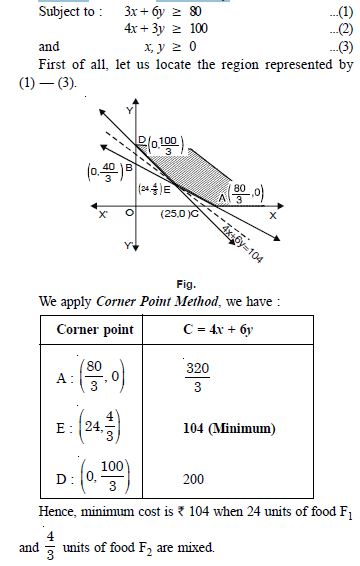##### Question 21:

There are two types of fertilizers F1 and F2. F1 consists of 10% nitrogen and 6% phosphoric acid and F2 consists of 5% nitrogen and 10% phosphoric acid. After testing the soil conditions, a farmer finds that she needs at least 14 kg. of nitrogen and 14 kg of phosphoric acid for her crop. If F1 costs ₹ 6/kg and F2 costs ₹ 5/kg, determine how much of each type of fertlizer should be used so that nutrient requirements are met at a minimum cost. What is the minimum cost ?

Let ‘x’ kg. of fertilizer F1 and ‘y’ kg. of fertilizer
F2 be required.
We have :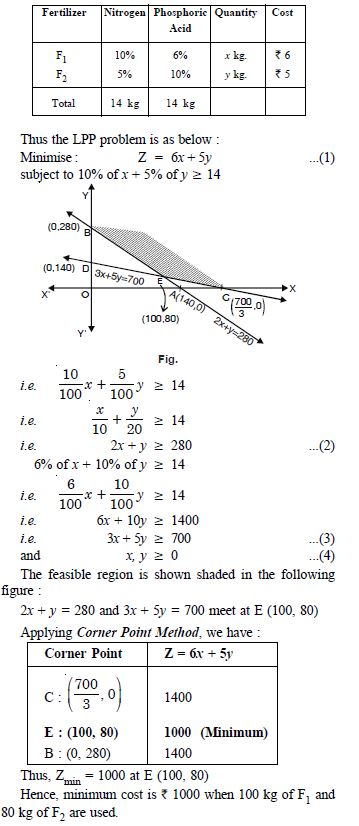##### Question 22:

The corner points of the feasible region determined by the following system of linear inequalities :
2x + y $\le$ 10, x + 3y $\le$ 15, x, y $\ge$0 are (0, 0), (5, 0), (3, 4) and (0, 5)
Let Z = px + qy, where p, q > 0. Condition on p and q so that the maximum of Z occurs at both (3, 4) and (0, 5) is :

1. p = q
2. p = 2q
3. p = 3q
4. q = 3p

q = 3p

##### Question 23:

The point, which does not lie in the half-plane 2x + 3y– 12 $\le$ 0 is:

1. (1, 2)
2. (2, 1)
3. (2, 3)
4. (2, 3)

(2, 3)

##### Question 24:

The point, which does not lie in the half-plane 3x + 4y – 15 $\le$ 0 is:

1. (1, 2)
2. (2, 1)
3. (2, 2)
4. (3, 2).

(3, 2).

##### Question 25:

The point, which lies in the half-plane 2x + 3y – 12 $\ge$ 0 is:

1. (1, 2)
2. (2, 1)
3. (2, 2)
4. (2, 3).

(2, 3).

##### Question 26:

Maximize Z = x + 2y subject to x + y $\ge$ 5, x $\ge$ 0, y $\ge$ 0 is :

1. 5 at (0, 5)
2. 10 at (0, 5)
3. 5 at (5, 0)
4. 10 at (5, 10)

10 at (0, 5)

##### Question 27:

Maximize Z = 3x + 2y subject to x + y $\ge$ 8, x $\ge$ 0, y $\ge$ 0 is :

1. 16 at (0, 8)
2. 16 at (8, 0)
3. 8 at (16, 0)
4. 8 at (0, 16).

16 at (0, 8)

##### Question 28:

Maximize Z = 2x + 3y subject to x + 2y $\le$ 6, x $\ge$ 4, y $\ge$ 0 is :

1. 6 at (6, 0)
2. 6 at (0, 6)
3. 12 at (6, 0)
4. 12 at (0, 6).

12 at (6, 0)

##### Question 29:

The maximum value of Z = 3x + 4y subject to the constraints :
x + y $\le$4; x $\ge$0, y $\ge$0 is :

1. 0
2. 12
3. 16
4. 18

16

##### Question 30:

The corner point of the feasible region determined by the system of linear constraints are (0, 10), (5, 3), (15, 15), (0, 20) ? Let Z = px + qy, where p, q > 0. Condition on p and q so that the maximum of Z occurs at both points (15, 15) and (0, 20) is :

1. p = q
2. p = 2q
3. q = 2p
4. q = 3p.

q = 3p.

##### Question 31:

A linear function, which is minimized or maximized is called:

1. An objective function
2. An optimal function
3. An optimal function
4. A feasiable function

An objective function

##### Question 32:

Objective fuction of a LPP is :

1. a function to be optimized
2. a constraint
3. a relation between the variables
4. None of these.

a function to be optimized

##### Question 33:

The optimal value of the objective function is attained at :

1. the points given by the corners of feasible region
2. the points given by the intersection of inequalities
3. the points given by the intersection of inequalities
4. the points given by the intersection of inequalities